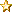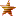###Author Topic: Interest rates are backward - should use APR  (Read 130 times)

#### meagher79

• Newbie
•• Posts: 12##### Interest rates are backward - should use APR
« on: May 22, 2020, 12:46:50 pm »
I just realized that the interest rate listed for loans is the total interest for the duration of the loan not the annual percentage rate (APR).  That means that 5 year loans actually have a much lower interest rate than 1 year loans!  There is no reason to ever take a shorter loan as it is.  An easy fix would be to change the label for "interest rate" to "total interest percentage" and then increase the interest on longer loans and/or decrease it for shorter loans.  Generally lenders require a higher rate for a longer loan because of the increased risk and because their money is tied up for longer.

#### patson

• Administrator
• Hero Member
•• Posts: 914##### Re: Interest rates are backward - should use APR
« Reply #1 on: May 27, 2020, 01:38:42 am »
Im not a loan expert here the rate we listed indeed in not APR

But im not sure if 5 years loan has much lower interest rate than 1 year lean? can you please explain it?

For example, if someone takes a \$100 loan, and the 1 year rate is 10% and 5 year rate is 15%. I would supposed that the on 1 year term the total amount to repay is \$110 and on 5 year term the total amount to replay is \$115, it is true that on 5 year term, on average only \$3 per year on interest, but at the same time the borrower really just getting \$20 loan on average per year over 5 years period?

#### meagher79

• Newbie
•• Posts: 12##### Re: Interest rates are backward - should use APR
« Reply #2 on: May 28, 2020, 12:24:27 pm »
The key point is that the interest is charged each year regardless of the length of the loan.  Let's take a very simple example using "simple interest" instead of compound interest.  A \$100 loan for one year at 10% interest would = \$110 total repayment as you said, but a \$100 loan at 10% interest for 5 years would = \$150 because there is 10% interest each year.

The situation you describe with the 5 year loan does not reflect the circumstances because the borrower gets the full \$100 at the beginning and gets to use the \$100 for five years (less the amount repaid over time which does reduce the interest).

Maybe think of the 5 year loan as a series of one year loans.  Suppose on Jan. 1 of 2010, I borrow \$100 to buy an airplane part.  On Jan. 1, 2011, I owe \$110, but I'm not ready to repay, so I pay \$10 interest, but I borrow another \$100 to repay the first loan.  The first loan is done, but on Jan 1, 2012 I owe \$110 for the second loan.  Again I get a new loan to repay the old one.  Each year I have to pay \$10 for the use of the \$100 I continue to have borrowed.  A five year loan just combines this process.  (Actually it gets more complicated depending on the repayment schedule and compounding interest.)

Try playing around with a loan calculator such as this one: https://www.calculator.net/loan-calculator.html
You are using the first option: Amortized Loan, where the principle is paid off gradually over the life of the loan.  As an example I looked at a \$100,000 loan for 260 months in game.  The total repayment was \$124,100 (which the game calls 24.1% interest rate, actually its the total interest)  I played around with the interest rate until I got the same total repayment and it was 8.85%

A very simple solution would be to just eliminate the shorter loans (they are a terrible deal compared to the 5yr), and change the "interest rate" label to "total interest percentage" as I suggested.  The actual 5 yr loan rate seems pretty reasonable.

#### meagher79

• Newbie
•• Posts: 12##### Re: Interest rates are backward - should use APR
« Reply #3 on: June 03, 2020, 10:47:49 am »
I will include an example from the game.  Right now I could borrow \$100,000 for one year at \$2,277 for 52 weeks.  This would be \$18,400 in total interest and a total repayment of \$118,400.  This is all good so far.  The problem is that the game calls this an 18.4% "interest rate."  In fact, the interest rate on this loan is much higher than 18.4% because the principle is being paid off over time, but the amount of interest charged doesn't go down.  I would be paying interest on money I have already paid back.  I tried to match the terms of this loan using the loan calculator and it is actually 34% APR.  Look at the top option, "Amortized Loan", at the link.  https://www.calculator.net/loan-calculator.html?cloanamount=100000&cloanterm=1&cloantermmonth=0&cinterestrate=34&ccompound=weekly&cpayback=weekly&x=70&y=22#amortized-result

On the other hand the 5 year loan overestimates interest rates for reasons I explained above.  I can also borrow \$100,000 for five years at \$487 for 260 weeks.  The game calls this 26.% interest, but it actually works out to be about 9.8% interest.  Again see the link and look at the top loan option "Amortized Loan."  https://www.calculator.net/loan-calculator.html?cloanamount=100000&cloanterm=5&cloantermmonth=0&cinterestrate=9.8&ccompound=weekly&cpayback=weekly&x=34&y=18#amortized-result

Conclusion:
1yr loan = 34% APR
5yr loan = 9.8% APR
The rates listed in-game are misleading.  It's not that big of a deal because the actual repayment amounts are accurate.  I love this game, and I only hope to be helpful.

#### patson

• Administrator
• Hero Member
•• Posts: 914##### Re: Interest rates are backward - should use APR
« Reply #4 on: June 09, 2020, 11:00:29 pm »
thanks for the explanations

Do the total interest charge makes sense in this game right now?

For the interest rate i was simply doing (total interest / borrowed amount)Is APR people use for mortgage too? i know people use that for car loans

#### meagher79

• Newbie
•• Posts: 12##### Re: Interest rates are backward - should use APR
« Reply #5 on: June 10, 2020, 01:30:36 am »

Do the total interest charge makes sense in this game right now?

Sort of, I think the actual interest on the 5 yr loans is reasonable, but the information in the "interest rate" column is inaccurate and misleading.

Is APR people use for mortgage too? i know people use that for car loans

Yes, APR is the standard way to measure all interest rates.